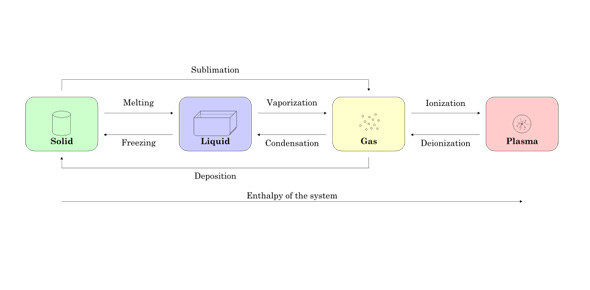# States Of Matter Test Quiz

24 Questions | Total Attempts: 580Settings• 1.
The process by which individual particles of a liquid escape from the surface and form a gas.
• A.

Melting point

• B.

Evaporation

• 2.
A liquid changing to a gas is ___.
• A.

Vaporization

• B.

Heat

• 3.
The ability to do work or cause change
• A.

Heat

• B.

Energy

• 4.
The kinetic and potential energy of the particles of a substance
• A.

Thermal energy

• B.

Boiing point

• 5.
Tells you whether a material is a solid, liquid, or gas
• A.

Freezing point

• B.

State of matter

• 6.
Anything that takes up space and has mass
• A.

Liquid

• B.

Matter

• 7.
Mass divided by volume
• A.

Density

• B.

Pressure

• 8.
Thermal energy that flows from higher temperature to lower temperature
• A.

Heat

• B.

Evaporation

• 9.
The temperature at which a substance changes from a solid to a liquid
• A.

Freezing point

• B.

Melting point

• 10.
The process by which gas particles move slowly enough for their attraction to bring them together to form a droplet of liquid
• A.

Vaporization

• B.

Condensation

• 11.
If the attractions between particles are strong enough to hold the particles in almost fixed positions but still slipping and sliding past each other, the matter involved is in a ____state
• A.

Solid

• B.

Liquid

• C.

Gas

• 12.
Solids are called ____ solids if their particles are arranged in a repeated three-dimensional pattern.
• A.

Amorphous

• B.

Chrystalline

• 13.
Because particles in a liquid move more freely than those in a solid, liquids have no definate
• A.

Shape

• B.

Volume

• 14.
A gas partially fills its container
• A.

True

• B.

False

• 15.
The physical state of a type of matter depends mostly upon how its atoms and molecules are arranged and how they move.
• A.

True

• B.

False

• 16.
The higher the temperature of matter the slower the particles are moving.
• A.

True

• B.

False

• 17.
Particles that make up matter are in a state of constant motion.
• A.

True

• B.

False

• 18.
When a warm object is brought near a cool object, the cool object will warm up.
• A.

True

• B.

False

• 19.
Vaporization is NOT a change from a liquid to a gas.
• A.

True

• B.

False

• 20.
If an object's density is less than that of the fluid it is in, it will float.
• A.

True

• B.

False

• 21.
The temperature remains constant when a liquid reaches its boiling point.
• A.

True

• B.

False

• 22.
A student measures the mass of an 8 cm3  block of brown sugar to be 12.9 g. What is the density of  the brown sugar?
• A.

16 g/cm cubes

• B.

.6 g/cm cubes

• C.

1.6 g/cm cubes

• D.

6 g/cm cubes

• 23.
A chef fills a 50 mL container with 43.5 g of cooking oil. What is the density of the oil? (Round to the nearest 10th)
• A.

1.1 g/ml

• B.

.9 g/ml

• C.

8 g/ml

• D.

9 g/ml

• 24.
A machine shop worker records the mass of an aluminum cube as 176 g. If one side of the cube  measures 4 cm, what is the density of the aluminum?
• A.

2.8 g/cm cubed

• B.

14.7g/cm cubed

• C.

.4g/cm cubed

• D.

44g/cm cubed

Related TopicsBack to top